## 一、隐马尔可夫模型

### 1.1HMM模型的定义

Q={q1,q2,...,qN},V={v1,v2,...vM}Q={q1,q2,…,qN},V={v1,v2,…vM}

I={i1,i2,...,iT},O={o1,o2,...oT}I={i1,i2,…,iT},O={o1,o2,…oT}

HMM模型做了两个很重要的假设如下：
1） 齐次马尔科夫链假设。即任意时刻的隐藏状态只依赖于它前一个隐藏状态。当然这样假设有点极端，因为很多时候我们的某一个隐藏状态不仅仅只依赖于前一个隐藏状态，可能是前两个或者是前三个。但是这样假设的好处就是模型简单，便于求解。如果在时刻tt的隐藏状态是it=qiit=qi,在时刻t+1t+1的隐藏状态是it+1=qjit+1=qj, 则从时刻t到时刻t+1的HMM状态转移概率aijaij可以表示为：

aij=P(it+1=qj|it=qi)aij=P(it+1=qj|it=qi)

A=[aij]N×NA=[aij]N×N

2） 观测独立性假设。即任意时刻的观察状态只仅仅依赖于当前时刻的隐藏状态，这也是一个为了简化模型的假设。如果在时刻t的隐藏状态是it=qjit=qj, 而对应的观察状态为ot=vkot=vk, 则该时刻观察状态vkvk在隐藏状态qjqj下生成的概率为bj(k)bj(k),满足：

bj(k)=P(ot=vk|it=qj)bj(k)=P(ot=vk|it=qj)

B=[bj(k)]N×MB=[bj(k)]N×M

Π=[π(i)]Nπ(i)=P(i1=qi)Π=[π(i)]N其中π(i)=P(i1=qi)

λ=(A,B,Π)λ=(A,B,Π)

### 1.2HMM模型的三个基本问题

HMM模型一共有三个经典的问题需要解决：
(1)评估观察序列概率。即给定模型λ=(A,B,Π)λ=(A,B,Π)和观测序列O={o1,o2,...oT}O={o1,o2,…oT}，计算在模型λ下观测序列O出现的概率P(O|λ)P(O|λ)。这个问题的求解需要用到前向后向算法。
(2)模型参数学习问题。即给定观测序列O={o1,o2,...oT}O={o1,o2,…oT}，估计模型λ=(A,B,Π)λ=(A,B,Π)的参数，使该模型下观测序列的条件概率P(O|λ)最大。这个问题的求解需要用到基于EM算法的鲍姆-韦尔奇算法。
(3)预测问题，也称为解码问题。即给定模型λ=(A,B,Π)λ=(A,B,Π)和观测序列O={o1,o2,...oT}O={o1,o2,…oT}，求给定观测序列条件下，最可能出现的对应的隐藏状态序列，这个问题的求解需要用到基于动态规划的维特比算法。

## 二、马尔科夫随机场

### 2.1条件独立性质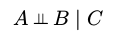###### 成对马尔可夫性（最大团的由来）：

P(Yu,Yv|Yo)=P(Yu|Yo)P(Yv|Yo)P(Yu,Yv|Yo)=P(Yu|Yo)P(Yv|Yo)
###### 局部马尔可夫性（马尔科夫毯）：

vVv∈V是无向图GG中任意一个节点，WW是与vv有边连接的所有节点，OOvWv、W以外的其他所有节点。v表示随机变量是YvWYv，W表示的随机变量组是YWYW，O表示的随机变量组是YoYo。局部马尔可夫性是指在给定随机变量组YWYW的条件下随机变量YvYv与随机变量组YoYo是独立的，即:

P(Yo,Yv|Yw)=P(Yv|Yw)P(Yo|Yw)P(Yo,Yv|Yw)=P(Yv|Yw)P(Yo|Yw)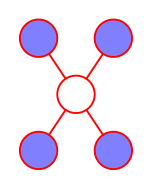###### 全局马尔可夫性：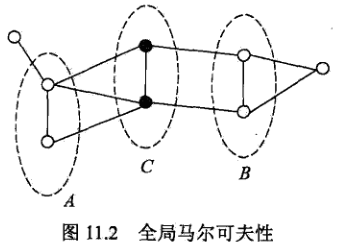P(YA,YB|YC)=P(YA|YC)P(YB|YC)P(YA,YB|YC)=P(YA|YC)P(YB|YC)

#### 2.3团Clique和因子

• 团( clique )：对于图中结点的一个子集，若其中任意两结点间都有边连接，则称该结点子集为一个团。
• 最大团( maximal clique )：若在一个团中加入另外任何一个结点都不再形成团，则称该团为极大团。### 2.4Hammersley-Clifford定理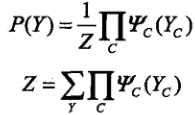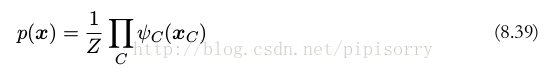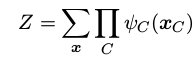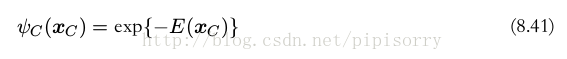## 三、条件随机场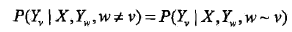### 3.1线性链条件随机场

X=(x1,x2,x3,xn),Y=(y1,y2,y3,yn)X=(x1,x2,x3⋯,xn),Y=(y1,y2,y3⋯,yn)均为线性链表示的随机变量序列，若在给定随机变量序列X的条件下，随机变量序列Y的条件概率分布P(Y|X)构成条件随机场，即满足马尔可夫性: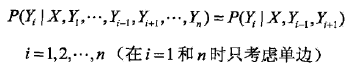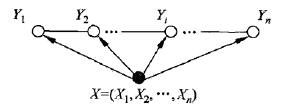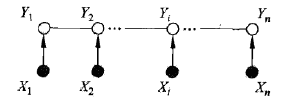X和Y有相同的图结构的线性链条件随机场

### 3.2条件随机场的不同形式

###### 3.2.1条件随机场的参数化形式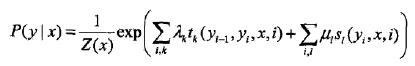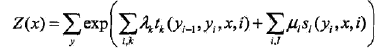（1）tktk是定义在边上的特征函数，称为转移特征，依赖于当前和前一个位置
（2）slsl是定义在及结点上的特征函数，称为状态特征，依赖于当前位置
（3）λkλk,μiμitktkslsl对应的权值
（4）特征函数tktkslsl取值为1或0：当满足特征条件时取值为1，否则为0。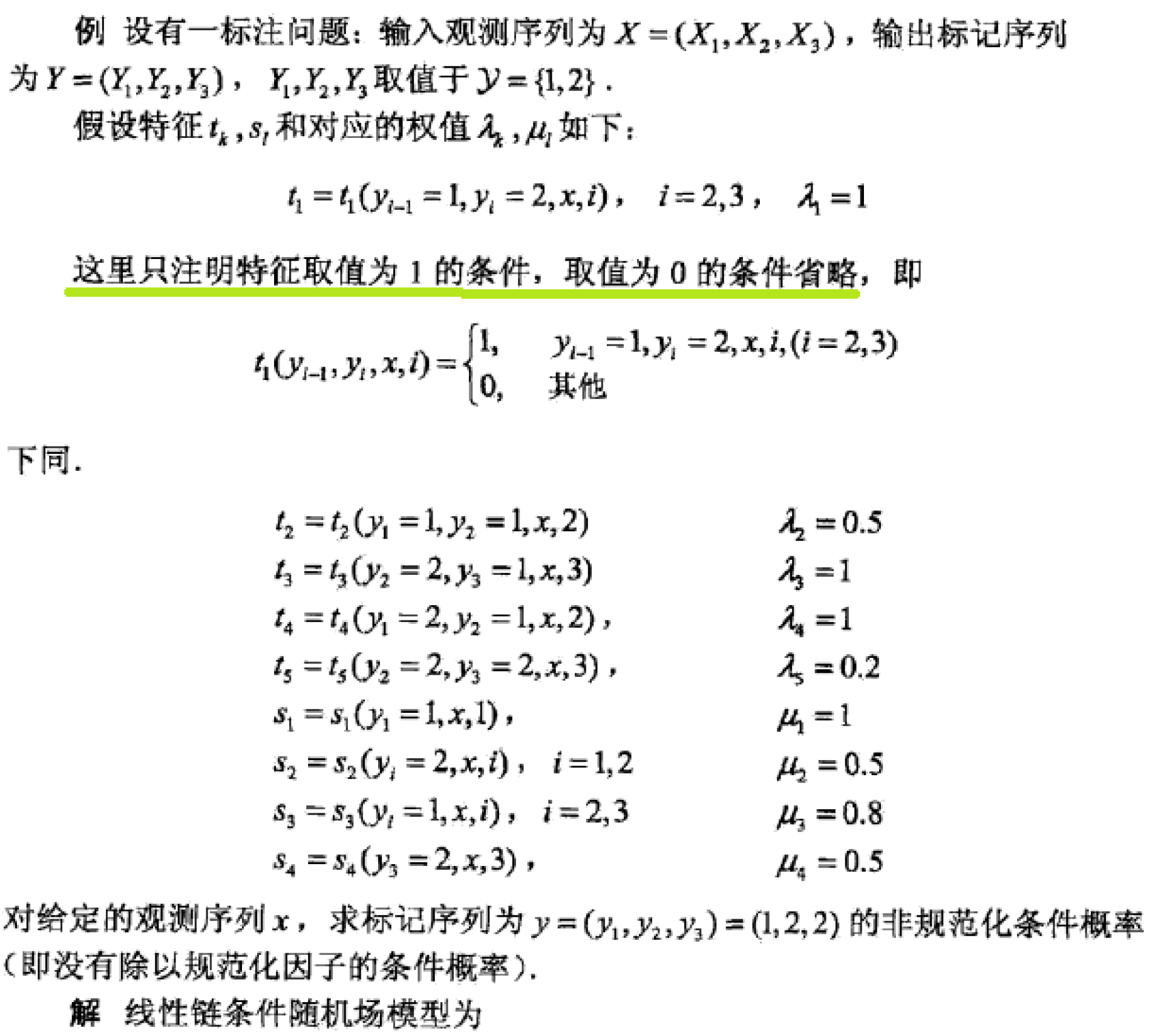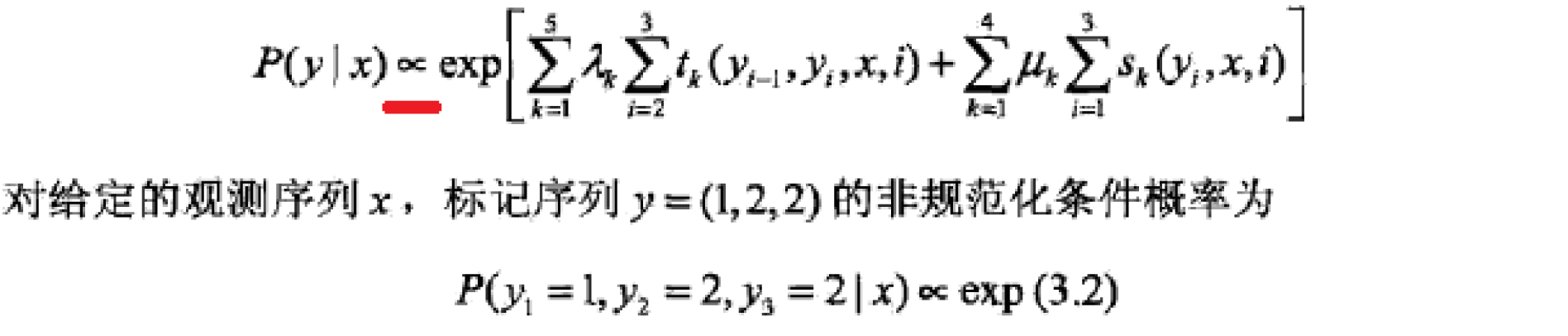ps:是3.2不是3：2